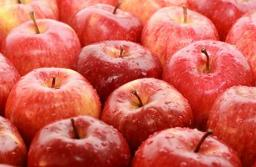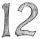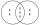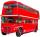# Apples 2

How many minimum apples are in the cart, if possible is completely divided into packages of 6, 14 and 21 apples?

x =  42

### Step-by-step explanation:Did you find an error or inaccuracy? Feel free to write us. Thank you!Tips to related online calculators
Do you want to calculate least common multiple two or more numbers?

## Related math problems and questions:

• CherriesCherries in the bowl can be divided equally among 3 or 15 or 5 children. How many are the minimum cherries in the bowl?
• Apples and pearsMom divided 24 apples and 15 pears to children. Each child received the same number of apples and pears - same number as his siblings. How many apples (j=?) and pears (h=?) received each child?
• Unknown integerFind the smallest integer: divided by 2, the remainder is 1. divided by 3, the remainder is 2. divided by 4, the remainder is 3. ... divided by eight, the remainder is 7, divided by 9 the remainder is 8.
• Lcm = 22 + gcdThe least common multiple of two numbers is 22 more than their greatest common divisor. Find these numbers.
• Gcd and lcmCalculate the greatest common divisor and the least common multiple of numbers. a) 16 and 18 b) 24 and 22 c) 45 and 60 d) 36 and 30
• LCM of two numberFind the smallest multiple of 63 and 147
• LCMWhat is the least common multiple of 5, 50, 14?
• Lcm of three numbersWhat is the Lcm of 120 15 and 5
• On Children'sOn Children's Day, the organizers bought 252 chewing gums, 396 candies and 108 lollipops. They want to make as many of the same packages as possible. Advise them what to put in each package and how many packages they can make this way.
• LCMCommon multiple of three numbers is 3276. One number is in this number 63 times, second 7 times, third 9 times. What are the numbers?
• Lcm to eliminate yIdentify the least common multiple that would eliminate the y-variable. 6x - 5y = -4 4x + 2y = 28
• LCD 2The least common denominator of 2/5, 1/2, and 3/4
• Lcm 2Create the smallest possible number that is divisible by numbers 5,8,9,4,3
• Buses 4intervals: 1st bus 40 min. 2nd bus 2h 3rd bud 20min How long take them to meet - as soon as possible?
• Lcm simpleFind least common multiple of this two numbers: 140 175.
• FractionsSort fractions z1 = (6)/(11); z2 = (10)/(21); z3 = (19)/(22) by its size. Result write as three serial numbers 1,2,3.
• PlumsIn the bowl are plums. How many would be there if we can divide it equally among 8, 10 and 11 children?